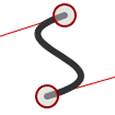How to Draw Bezier Curves on an HTML5 Canvas

In my last post, we created quadratic curves on HTML5 canvas elements using a few lines of code. If you examined the JavaScript on the quadratic curves demonstration page, you may have noticed several references to béziers — its curvier cousin.

What are bézier curves?

You’ve possibly seen bézier curves used in desktop publishing and graphics packages. So let’s take another trip to WolframMathWorld to look at the equations.

I’m sure you understood that but I’m feeling a little nauseous.In the last post, we saw how quadratic curves have a start point (P0), end point (P2) and a single control point (P1) which determines the curvature. In the case of canvas, a bézier curve has a start (P0), end (P3) and two control points — one for each end of the line (P1 and P2). Thanks again to Wikipedia for a great illustration.Béziers give far more control over the shape. Because the initial part of the curve follows its control path, we can generate curves which reverse direction or wrap on themselves. They are ideal for creating a Visio-like flow diagram where you need to join two shapes by a connector.

On to the code!

Like before, we require a few lines of JavaScript to get the canvas context and set the default widths and colors:

canvas = document.getElementById("canvas");
ctx = canvas.getContext("2d")
ctx.lineWidth = 6;
ctx.strokeStyle = "#333";

We now define the starting point for our curve (P0):

ctx.beginPath();
ctx.moveTo(100, 250);

The bezierCurveTo() method is then used to draw the curve. It requires six arguments:

• the x co-ordinate of the curve start control point (P1)
• the y co-ordinate of the curve start control point (P1)
• the x co-ordinate of the curve end control point (P2)
• the y co-ordinate of the curve end control point (P2)
• the x co-ordinate of the end point (P3)
• the y co-ordinate of the end point (P3)

Finally, we call the stroke() method to complete our curve:

ctx.bezierCurveTo(150, 100, 350, 100, 400, 250);
ctx.stroke();

The interactive canvas bézier curve demonstration page allows you to drag all the points and generate the code (sorry IE fans — you’ll need to wait for IE9).

I hope you found this series useful. Are you using canvas curves for any interesting projects?